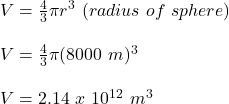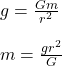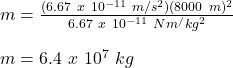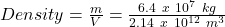Question

Find the density of a planet with a radius of 8000 m if the gravitational acceleration for the planet, gp, has the same magnitude as the gravitational constant, G (keep the right units for both), where G = 6.67 x 10-11 m3/(kg s2) Hint: Use the expression for the gravitational force and Newton’s second law.

1.Density = 3 x 10⁻⁵ kg/m³

Explanation:

First, we will find the volume of the planet:Now, we will use the expression for gravitational force to find the mass of the planet:where,

m = mass = ?

g = acceleration due to gravity = 6.67 x 10⁻¹¹ m/s²

G = Universal Gravitational Constant = 6.67 x 10⁻¹¹ Nm²/kg²

r = radius = 8000 m

Therefore,Therefore, the density will be:Density = 3 x 10⁻⁵ kg/m³